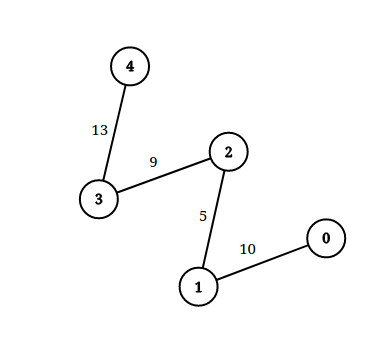1697. Checking Existence of Edge Length Limited Paths
Hard
1.8K
43

An undirected graph of `n` nodes is defined by `edgeList`, where `edgeList[i] = [ui, vi, disi]` denotes an edge between nodes `ui` and `vi` with distance `disi`. Note that there may be multiple edges between two nodes.

Given an array `queries`, where `queries[j] = [pj, qj, limitj]`, your task is to determine for each `queries[j]` whether there is a path between `pj` and `qj` such that each edge on the path has a distance strictly less than `limitj` .

Return a boolean array `answer`, where `answer.length == queries.length` and the `jth` value of `answer` is `true` if there is a path for `queries[j]` is `true`, and `false` otherwise.

Example 1:```Input: n = 3, edgeList = [[0,1,2],[1,2,4],[2,0,8],[1,0,16]], queries = [[0,1,2],[0,2,5]]
Output: [false,true]
Explanation: The above figure shows the given graph. Note that there are two overlapping edges between 0 and 1 with distances 2 and 16.
For the first query, between 0 and 1 there is no path where each distance is less than 2, thus we return false for this query.
For the second query, there is a path (0 -> 1 -> 2) of two edges with distances less than 5, thus we return true for this query.
```

Example 2:```Input: n = 5, edgeList = [[0,1,10],[1,2,5],[2,3,9],[3,4,13]], queries = [[0,4,14],[1,4,13]]
Output: [true,false]
Explanation: The above figure shows the given graph.
```

Constraints:

• `2 <= n <= 105`
• `1 <= edgeList.length, queries.length <= 105`
• `edgeList[i].length == 3`
• `queries[j].length == 3`
• `0 <= ui, vi, pj, qj <= n - 1`
• `ui != vi`
• `pj != qj`
• `1 <= disi, limitj <= 109`
• There may be multiple edges between two nodes.
Accepted
45.8K
Submissions
72.6K
Acceptance Rate
63.0%

Seen this question in a real interview before?
1/4
Yes
No

Discussion (0)

Related Topics